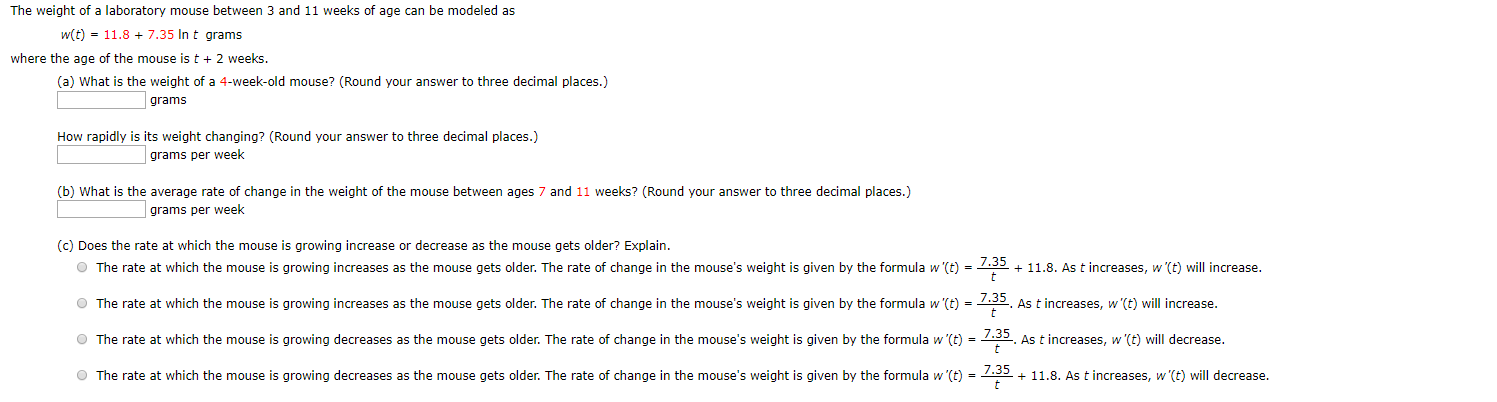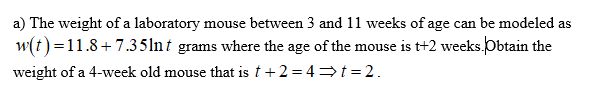# The weight of a laboratory mouse between 3 and 11 weeks of age can be modeled asw(t) 11.8+7.35 In t gramswhere the age of the mouse is t 2 weeks.(a) What is the weight of a 4-week-old mouse? (Round your answer to three decimal places.)gramsHow rapidly is its weight changing? (Round your answer to three decimal places.)grams per week(b) What is the average rate of change in the weight of the mouse between ages 7 and 11 weeks? (Round your answer to three decimal places.)grams per week(c) Does the rate at which the mouse is growing increase or decrease as the mouse gets older? Explain.OThe rate at which the mouse is growing increases as the mouse gets older. The rate of change in the mouse's weight is given by the formula w '(t) = --11.8. As t increases, w (t) will increase...35OThe rate at which the mouse is growing increases as the mouse gets older. The rate of change in the mouse's weight is given by the formula w '(t) =As t increases, w'(t) will increase.The rate at which the mouse is growing decreases as the mouse gets older. The rate of change in the mouse's weight is given by the formula w'(t) .35 As tincreases, w'(t) will decrease.tO The rate at which the mouse is growing decreases as the mouse gets older. The rate of change in the mouse's weight is given by the formula w'(t)11.8. As t increases, w'(t) will decrease.t

Questionhelp_outlineImage TranscriptioncloseThe weight of a laboratory mouse between 3 and 11 weeks of age can be modeled as w(t) 11.8+7.35 In t grams where the age of the mouse is t 2 weeks. (a) What is the weight of a 4-week-old mouse? (Round your answer to three decimal places.) grams How rapidly is its weight changing? (Round your answer to three decimal places.) grams per week (b) What is the average rate of change in the weight of the mouse between ages 7 and 11 weeks? (Round your answer to three decimal places.) grams per week (c) Does the rate at which the mouse is growing increase or decrease as the mouse gets older? Explain. OThe rate at which the mouse is growing increases as the mouse gets older. The rate of change in the mouse's weight is given by the formula w '(t) = -- 11.8. As t increases, w (t) will increase. ..35 OThe rate at which the mouse is growing increases as the mouse gets older. The rate of change in the mouse's weight is given by the formula w '(t) = As t increases, w'(t) will increase. The rate at which the mouse is growing decreases as the mouse gets older. The rate of change in the mouse's weight is given by the formula w'(t) .35 As tincreases, w'(t) will decrease. t O The rate at which the mouse is growing decreases as the mouse gets older. The rate of change in the mouse's weight is given by the formula w'(t) 11.8. As t increases, w'(t) will decrease. t fullscreen
check_circleExpert Solution
Step 1Step 2

Obtain the rate at which the weight changes as,

Step 3

Therefore, the rate at which the weight ...

### Want to see the full answer?

See Solution

#### Want to see this answer and more?

Solutions are written by subject experts who are available 24/7. Questions are typically answered within 1 hour*

See Solution
*Response times may vary by subject and question
Tagged in

### Calculus